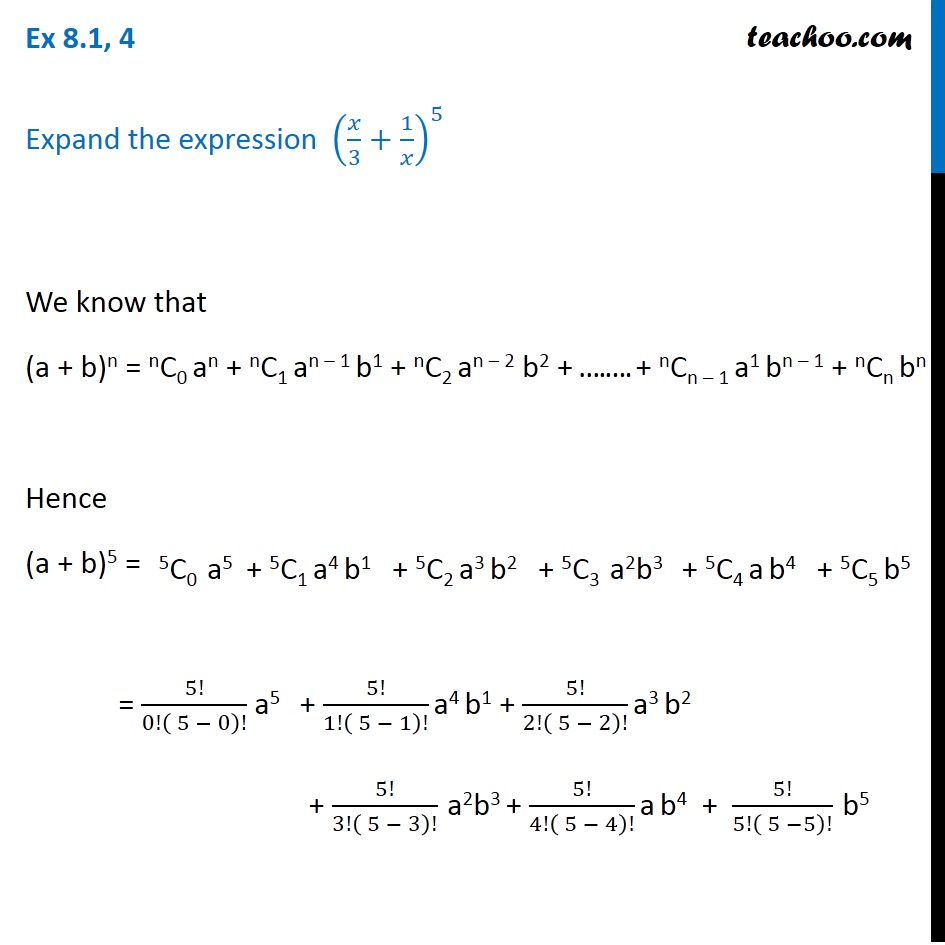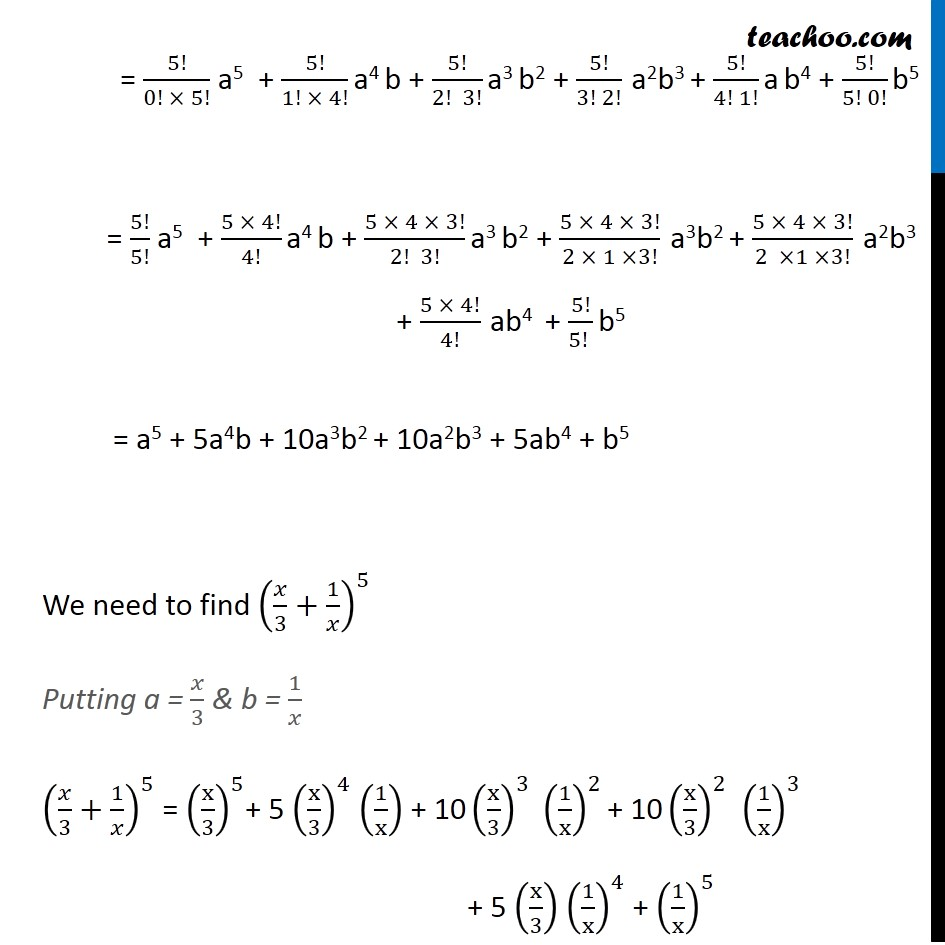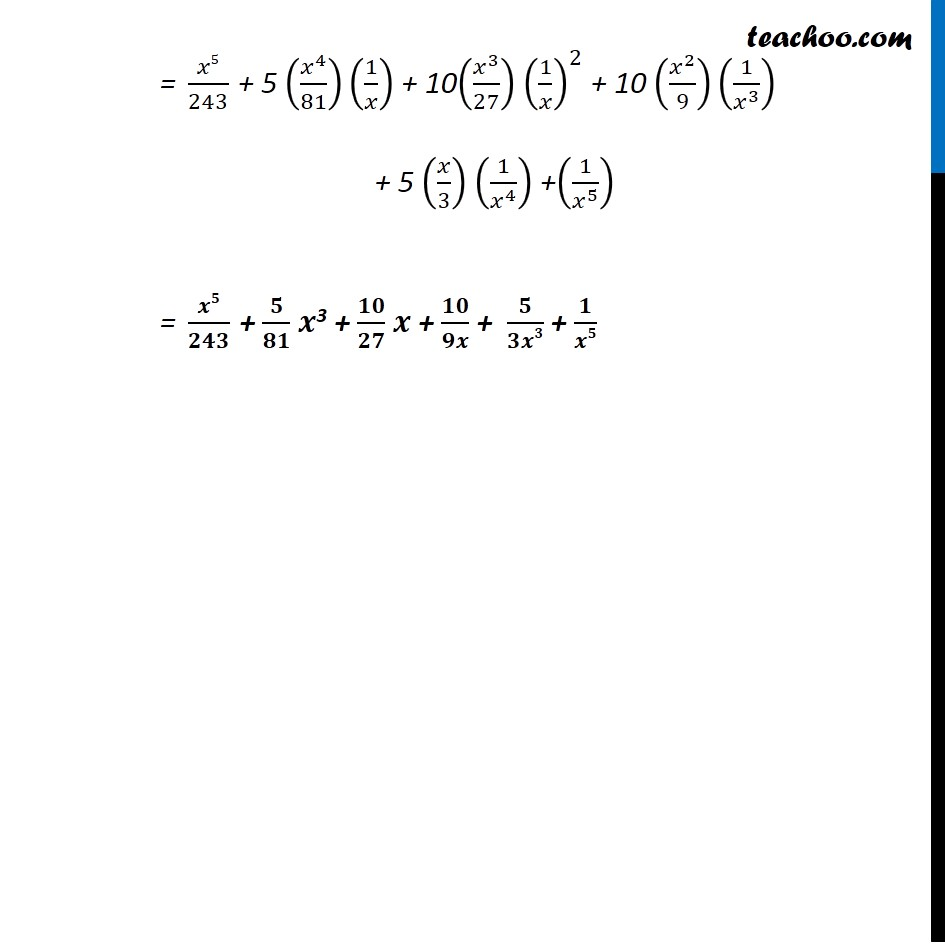Ex 7.1

Chapter 7 Class 11 Binomial Theorem
Serial order wiseLearn in your speed, with individual attention - Teachoo Maths 1-on-1 Class

### Transcript

Ex 7.1, 4 Expand the expression (𝑥/3+1/𝑥)^5 We know that (a + b)n = nC0 an + nC1 an – 1 b1 + nC2 an – 2 b2 + ….…. + nCn – 1 a1 bn – 1 + nCn bn Hence (a + b)5 = = 5!/0!( 5 − 0)! a5 + 5!/1!( 5 − 1)! a4 b1 + 5!/2!( 5 − 2)! a3 b2 + 5!/3!( 5 − 3)! a2b3 + 5!/4!( 5 − 4)! a b4 + 5!/5!( 5 −5)! b5 = 5!/(0! × 5!) a5 + 5!/(1! × 4!) a4 b + 5!/(2! 3!) a3 b2 + 5!/(3! 2!) a2b3 + 5!/(4! 1!) a b4 + 5!/(5! 0!) b5 = 5!/5! a5 + (5 × 4!)/4! a4 b + (5 × 4 × 3!)/(2! 3!) a3 b2 + (5 × 4 × 3!)/(2 × 1 ×3!) a3b2 + (5 × 4 × 3!)/(2 ×1 ×3!) a2b3 + (5 × 4!)/4! ab4 + 5!/(5! ) b5 = a5 + 5a4b + 10a3b2 + 10a2b3 + 5ab4 + b5 We need to find (𝑥/3+1/𝑥)^5 Putting a = 𝑥/3 & b = 1/𝑥 (𝑥/3+1/𝑥)^5 = (x/3)^5+ 5 (x/3)^4 (1/x) + 10 (x/3)^3 (1/x)^2 + 10 (x/3)^2 (1/x)^3 + 5 (x/3) (1/x)^4 + (1/x)^5 = 𝑥5/243 + 5 (𝑥^4/81)(1/𝑥) + 10(𝑥^3/27) (1/𝑥)^2 + 10 (𝑥^2/9)(1/𝑥^3 ) + 5 (𝑥/3) (1/𝑥^4 ) +(1/𝑥^5 ) = 𝒙𝟓/𝟐𝟒𝟑 + 𝟓/𝟖𝟏 𝒙3 + 𝟏𝟎/𝟐𝟕 𝒙 + 𝟏𝟎/𝟗𝒙 + 𝟓/𝟑𝒙𝟑 + 𝟏/𝒙𝟓